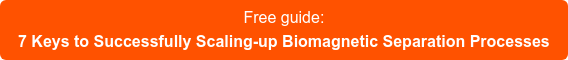Blog

To successfully scale up a biomagnetic separation process is necessary to understand the key parameter governing it. To move a magnetic bead we need to apply a magnetic force over it. This force would make the bead move in a direction and be in equilibrium with the drag force generated by the viscosity of the buffer. The result would be a constant velocity (if the magnetic force is constant).The first usual misunderstanding is the assumption that the force would be proportional to the magnetic field. Checking any physics handbook you will notice that a uniform magnetic force DOES NOT generate a magnetic force. A uniform magnetic field will only make the beads rotate, not move from their position. The confusion between magnetic force and magnetic field happens since generating a perfectly uniform magnetic is difficult. A permanent magnet generates a field varying on the space, and it's this variation the real cause of the magnetic force responsible of the magnetic beads displacement. However, as stronger magnets generate stronger gradients, many people interpret the observation as a direct correlation between force and field.

By taking a closer look to the expression of the magnetic force we realize that we need to take into account how it varies with the magnetic field and also consider the magnetic moment of the bead. The magnetic moment of magnetic beads or particles typically varies at low fields and is saturated at high fields. Thus, the expression of the force is different in both field regions. When the main characteristic is of constant susceptibility (the magnetic moment changes proportionally to the applied field), the magnetic force is proportional to the spatial variation of the square of the magnetic field. When the magnetic beads are saturated (i.e. the magnetic moment is constant), the magnetic force is proportional to the gradient of the magnetic moment.Knowing the factors controlling the magnetic force is key for understanding the biomagnetic separation process, defining the right procedures and correctly validating the operational procedures.

Related post: